Starts With A Bang

# Why 28 + 47 = 72, Not 75, For Black Holes

#### Even addition has to play by different rules for black holes.

How do you add 28 and 47 together? This simple math question helps us highlight the many different ways that people conceptualize numbers in their heads. Some of us break down 28 and 47 into 20 + 8 and 40 + 7, and then go from there. Equivalently, you can view them as 30–2 and 50–3, and then combine those results. Another approach is to split them into 25 + 3 and 50–3, with many other possible, and equivalent, approaches. As long as your methods are sound and you get the right answer, that 28 + 47 = 75, there isn’t really a wrong way to do it.

But for certain physical objects obeying the law of gravity, addition isn’t always so simple. If you merged a 28 solar mass black hole with a 47 solar mass black hole, the black hole you wind up with, at the end, would be 72 solar masses, not 75. In fact, for any two black holes that you merge together, you wind up with less mass than you started with. This isn’t due to a flaw in our math, but rather something very special about how gravity works. Here’s why merging black holes always lose mass.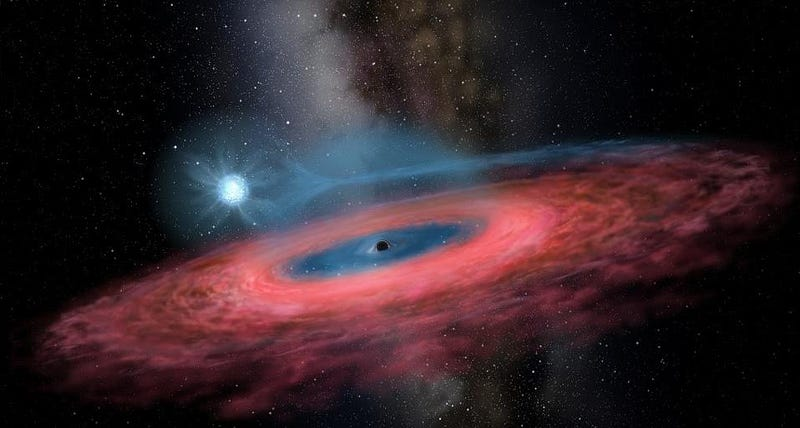When a black hole and a companion star orbit one another, the star’s motion will change over time owing to the gravitational influence of the black hole, while matter from the star can accrete onto the black hole, resulting in X-ray and radio emissions. If another black hole is orbiting instead, gravitational radiation will dominate. (JINGCHUAN YU/BEIJING PLANETARIUM/2019)

One of the first scientific rules we learn in our lives is the conservation of energy. It tells us that energy can never be created or destroyed, but only converted from one form into another. If you lift up a heavy block, you must do work (a form of energy) against the force of gravity: you input energy into the block. As a result, the block gains gravitational potential energy. When you drop the block, that potential energy gets converted into kinetic energy, and at the instant the block hits the floor, that energy gets converted into a variety of other forms: heat, deformation, and sonic energy, among others.

When you start with two masses, therefore, there’s a specific amount of total energy that must be present as well: the energy inherent to anything with mass, given by Einstein’s most famous equation, E = mc². There are, of course, other forms of energy as well, and three of them can’t be ignored. Two of them are more obvious than the third, but we have to consider all the relevant forms of energy if we want to make sure that everything that needs to be conserved actually is.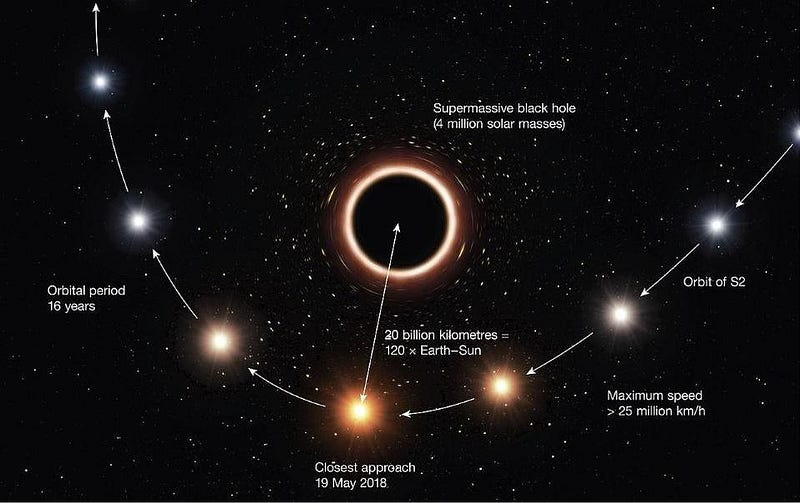Owing to the effects of both its high velocity (Special Relativity) and the curvature of space (General Relativity), a star passing close to a black hole should undergo a number of important effects, which will translate into physical observables like the redshifting of its light and a slight but significant alteration of its elliptical orbit. The close approach of S0–2 in May of 2018 was the best chance we’ve gotten to examine these relativistic effects and scrutinize Einstein’s predictions. (ESO/M. KORNMESSER)

In addition to rest mass energy, the three types of energy we need to consider are as follows.

1.) There’s gravitational potential energy, which is determined by how distant these two masses are from one another. Masses that are an infinite distance away from each other have zero gravitational potential energy, while the closer they get to one another, the more “deformed” spacetime will be, and hence we’ll get a large and negative amount of gravitational potential energy.

2.) There’s kinetic energy, which is determined by the relative motion of these two masses to one another. The faster you move, the greater your kinetic energy. The combination of kinetic and potential energy explains why “falling” objects speed up: as your gravitational potential energy gets more and more negative, it turns into larger and larger positive kinetic energies.

3.) And there’s the energy in gravitational waves, a form of gravitational radiation that carries energy away from a system.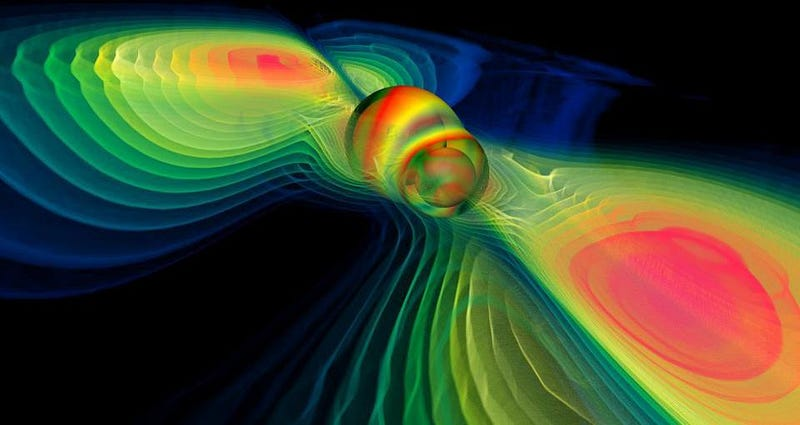When two objects inspiral or merge, they produce enormous amounts of gravitational waves. Simply traveling through curved space is a great way to get massive particles to radiate gravitationally: a fundamental difference between Einstein’s and Newton’s gravity. (WERNER BENGER, CC BY-SA 4.0)

While rest mass energy, gravitational potential energy, and kinetic energy are all concepts that work perfectly well with Newtonian mechanics and gravitation, the idea of gravitation radiation is inherently novel in Einstein’s General Relativity. When a mass moves through a region of space where the underlying spacetime curvature changes, or where a mass accelerates (changing direction) even when the spacetime curvature remains constant, the interaction causes the emission of a specific type of radiation: gravitational waves.

Any mass that orbits any other mass will emit it, with the smaller mass typically experiencing the largest effects. For example, we think of Earth being in a stable orbit around the Sun, but that’s not quite technically true. If the Sun were to keep its properties constant — no changes in mass, ever — the Earth would not remain in an elliptical orbit forever. Rather, the planets would slowly radiate energy away, their orbits would decay, and they would eventually spiral into the Sun. It might take Earth some ~10²⁶ years to succumb to this fate, an unobservably long time, but if gravitational radiation is real, this decay will occur.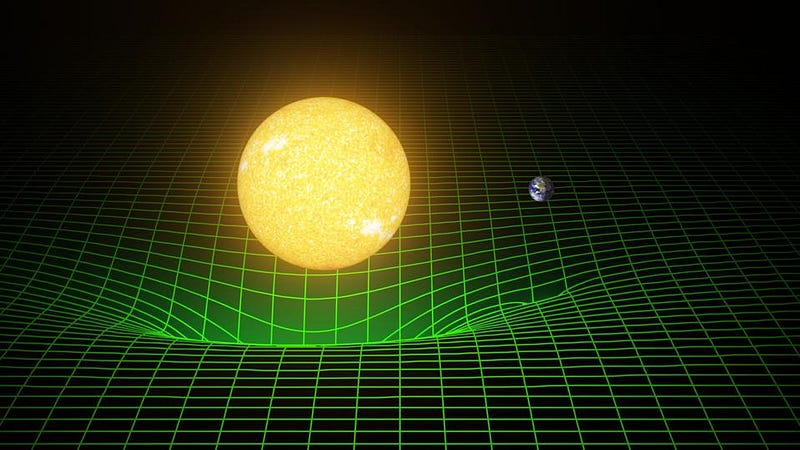The gravitational behavior of the Earth around the Sun is not due to an invisible gravitational pull, but is better described by the Earth falling freely through curved space dominated by the Sun. The shortest distance between two points isn’t a straight line, but rather a geodesic: a curved line that’s defined by the gravitational deformation of spacetime. As it travels through this curved space, the Earth emits gravitational waves. (LIGO/T. PYLE)

Many astrophysical scenarios exist, however, where the effects of gravitational waves are far more pronounced. In general, any effect that only exists in General Relativity (and not in Newtonian gravity) will be strongest where:

• masses are large,
• distances are small,
• and the curvature of space is large.

Where do we have large masses at small distances where the spatial curvature is highly significant? Near massive, compact objects: white dwarfs, neutron stars, and black holes. Of all of these, black holes have the largest masses, the smallest volumes, can be approaches at the closest distances, and exhibit the largest amounts of spatial curvature.

But black holes are extremely difficult to detect and observe, whereas many neutron stars have a telltale signature: they pulse very regularly. When one pulsing neutron star orbits another large mass — like another neutron star or a black hole — we can start to measure how these pulses behave, and they reveal something fascinating.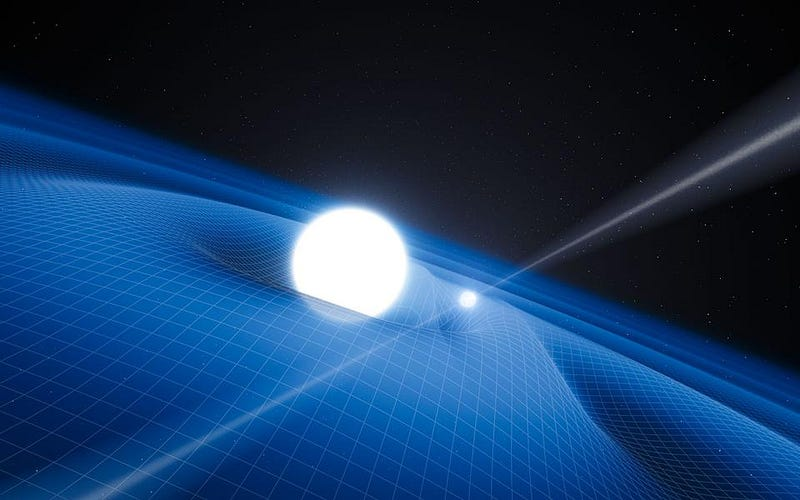A pulsar with a massive binary companion, particularly a compact companion like a white dwarf, another neutron star, or a black hole, can emit significant amounts of gravitational waves. This emission will cause a change in the pulsar timing observations, leading to a test of relativity. (ESO/L. CALÇADA)

If the neutron star were in a perfectly stable orbit, not decaying in any way due to the emission of the predicted gravitational waves, the pattern of pulses we’d receive would be constant with time. If the orbit was decaying, however, we would see that pulse pattern evolve, and in particular we’d see the orbit itself start to speed up. (When you lose energy, you fall closer in towards the other masses, and that means tighter, faster orbits.)

Since the 1960s, we’ve known about binary pulsars: pulsars that orbit another neutron star. We’ve also known about “singlet” pulsars, or pulsars that are the only large mass in their system. What do we find, with long-term observations of these objects? That singlet pulsars have a very consistent pattern of pulses, and that pattern doesn’t evolve over time. But for binary pulsars, not only do we witness a changing pattern in the pulses we observe, but that pattern changes in exactly the fashion predicted by General Relativity from the emission of gravitational waves.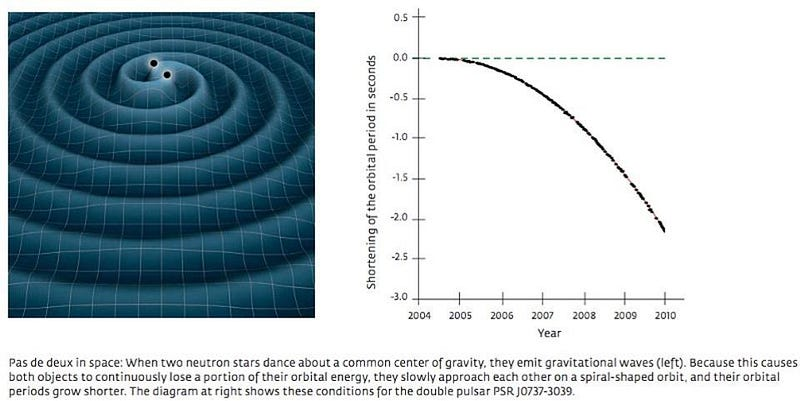Relativistic prediction (red line) and Newtonian (green) versus the binary pulsar data (black). From the very first binary neutron star system ever discovered, we knew that gravitational radiation was carrying energy away. It was only a matter of time before we found a system in the final stages of inspiral and merger. (NASA (L), MAX PLANCK INSTITUTE FOR RADIO ASTRONOMY / MICHAEL KRAMER)

Although neutron stars can be both massive and incredibly compact — reaching masses of up to a little over 2 solar masses and with sizes of just ~10-to-20 kilometers — black holes are even more extreme. Their masses are compressed down to a singularity, hidden behind an event horizon, where only their mass and angular momentum determine the size and shape of the horizon: the boundary between where anything can and cannot theoretically escape from it.

When a black hole orbits another one, in what’s known as a binary black hole system, each mass experiences the effects of the curved spacetime from the other one. As they mutually orbit one another, the mass and the curved spacetime interact, causing the emission of radiation. (An analogous effect happens in electromagnetism, where a charged particle moving/accelerating through a changing electromagnetic field emits radiation.) The magnitude of the masses, the separation of the masses, and the speed of the masses moving through that curved spacetime determines the amplitude, frequency, and energy emitted through gravitational radiation.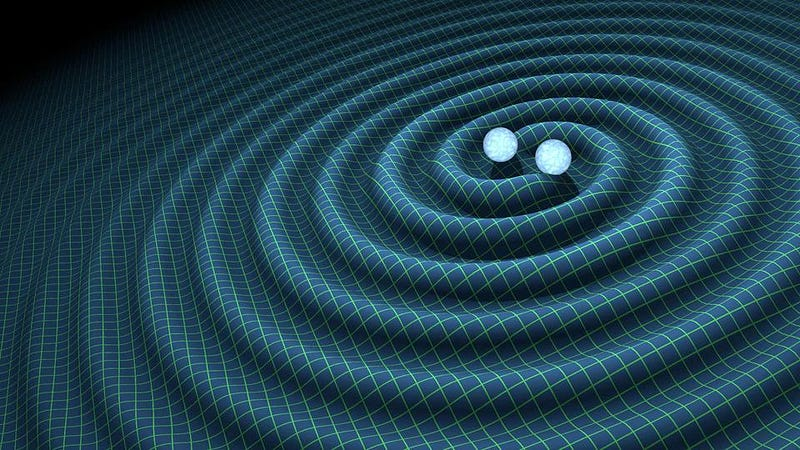The ripples in spacetime form orbiting masses will occur irrespective of what the ultimate merger product is. However, the majority of the energy released comes from just the final few orbits and the actual merger of the two masses that inspiral and merge. (R. HURT — CALTECH/JPL)

What might be surprising is that the overwhelming majority of the energy emitted — something like 90% or more — happens during just the final two or three orbits of these masses around one another, as well as at the moment of the merger itself. If it weren’t for this energy peak at the very end of a long, cosmic dance, we would have entirely missed many of the gravitational wave events we’ve seen, including the very first one.

In many cases, it’s only the spike of these final milliseconds that provides us with the surefire signature of a gravitational wave signal rising above the noise. (The remaining signal is often also extracted.) In many ways, the gravitational wave events we see are the most energetic ones to occur since the Big Bang. For example, over the final few milliseconds, where up to a handful of solar masses can be converted into gravitational wave energy, a single black hole-black hole merger can emit more energy than all the stars in the Universe combined.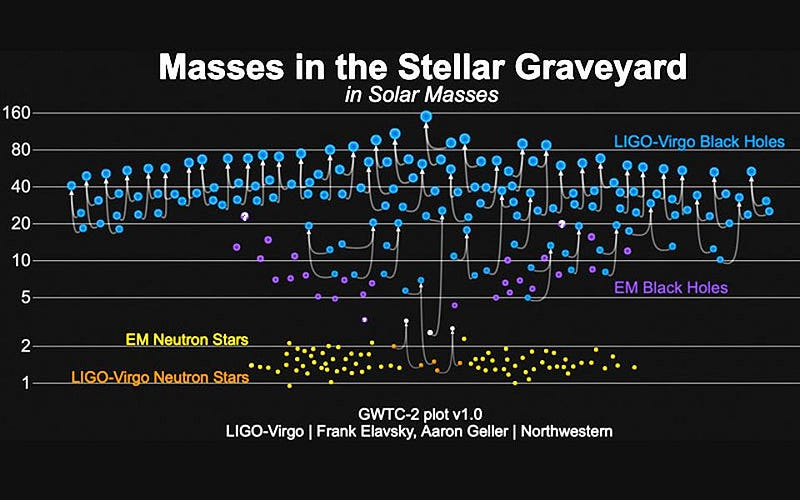This plot shows the masses of all compact binaries detected by LIGO/Virgo, with black holes in blue and neutron stars in orange. Also shown are stellar mass black holes (purple) and neutron stars (yellow) discovered with electromagnetic observations. All told, we have more than 50 observations of gravitational wave events corresponding to compact mass mergers. (LIGO/VIRGO/NORTHWESTERN UNIV./FRANK ELAVSKY)

One of the thing that’s fun about this is that there’s a simple approximation you can use to answer the question of, “for any two black holes that merge, how much mass gets converted into energy?”

The approximation? Just take the smaller of the two merging black hole masses, multiply it by 0.1, and that’s how much mass, roughly, gets converted into energy. That’s right: 10% of the smaller mass black hole.

There are all sorts of complicated effects at play, and a large rotational component to a black hole — which many of them have — can slightly change the story. But the effects of mass are generally dominant over spin/angular momentum, and the effects of having lopsided mass ratios is generally small. In fact, physicist Vijay Varma went and constructed a graph that tested this approximation for a variety of mass ratios, and as you can see, “10% of the smaller mass” is an excellent approximation for how much mass gets converted to energy when two black holes merge.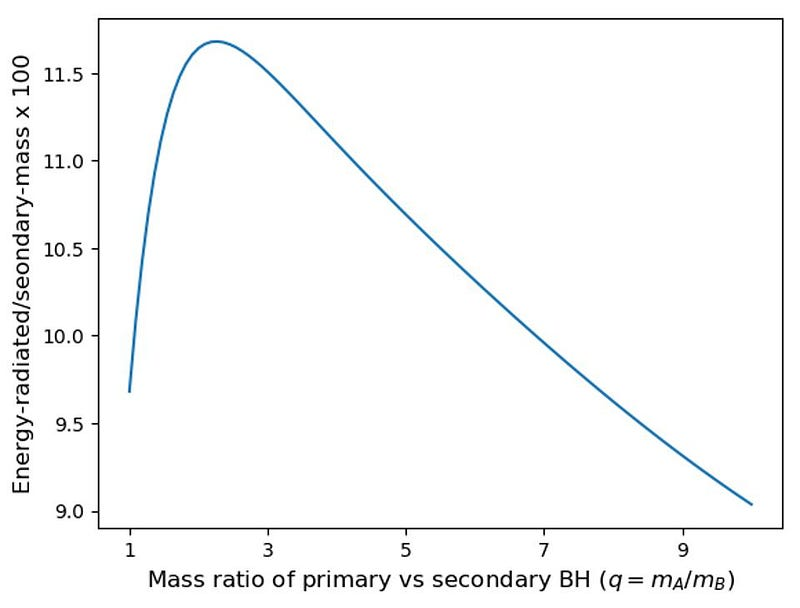How much mass gets converted into gravitational waves when two black holes merge. Note that although the graph appears to show large variations as a function of mass ratios, the y-axis scale is very small, and that “10%” makes a good approximation across a wide range of mass ratios. (VIJAY VARMA)

If you ever have two black holes merging and you know their initial masses, you can predict how much of those masses will become a final, post-merger black hole, and how much will be radiated away in the form of gravitational waves. Just take the smaller mass black hole, take 10% of that mass away, and the remainder combines with the other black hole to make your final one. Meanwhile, that “10% of the smaller mass black hole” gets converted into gravitational waves, where it will travel the Universe in all directions.Travel the Universe with astrophysicist Ethan Siegel. Subscribers will get the newsletter every Saturday. All aboard!

So if you have black holes of 46 and 40 solar masses, your final black hole will be 82 solar masses, with 4 solar masses radiated away.

If they’re 53 and 10 solar masses, your final black hole will be 62 solar masses, with 1 solar mass radiated away.

And if they’re 47 and 28 solar masses, your final black hole will be 72.2 solar masses, with 2.8 solar masses radiated away.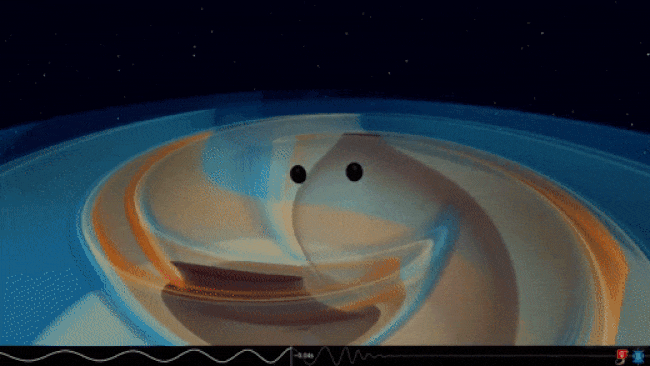Two black holes of approximately equal mass, when they inspiral and merge, will exhibit the gravitational wave signal (in amplitude and frequency) shown at the bottom of the animation. The gravitational wave signal will spread out in all three dimensions at the speed of light, where it can be detected from billions of light-years away by a sufficient gravitational wave detector. (N. FISCHER, H. PFEIFFER, A. BUONANNO (MAX PLANCK INSTITUTE FOR GRAVITATIONAL PHYSICS), SIMULATING EXTREME SPACETIMES (SXS) COLLABORATION)

As long as space is curved and you have mass, you can’t move through it without emitting gravitational radiation. In the most severe cases of all, it even affects the way you do addition. It took 100 years from the first prediction of gravitational waves until the first direct measurement of them, and that achievement has never looked more spectacular. As our observations improve, we’ll be able to pin down more subtle effects superimposed atop this simple approximation. But for now, enjoy the simplicity of the black hole math that everyone can do!

Starts With A Bang is written by Ethan Siegel, Ph.D., author of Beyond The Galaxy, and Treknology: The Science of Star Trek from Tricorders to Warp Drive.

Related
The cathedral is being explored as never before.
It’s more than just a cute habit.
“Not my circus, not my monkeys.”
What makes some scientists culturally significant, while others remain in obscurity? Well, there’s a science to it.
9 min
with
Explanations for the cosmic speed limit often conflate mass with inertia.

Up Next
A machine learning system lets visitors at a Kandinsky exhibition hear the artwork.